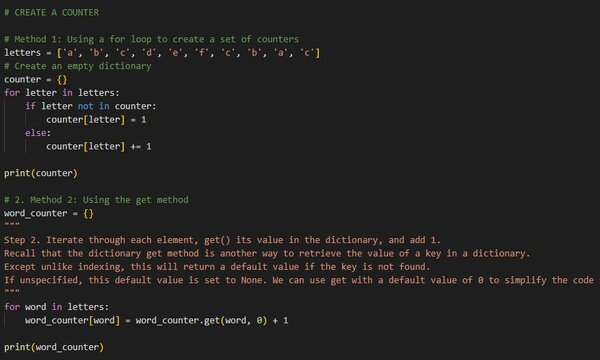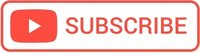# Conditional Statements in Python## If / Else / Elif Statements

### If Statement

If statement runs or skips the code based on whether a condition is true or false.

For conditions, You can use simple operators (<, >, ==) or combine them with and, or and not together. Use parentheses if you need to make the combinations clear.

num1 = 5
num2 = 10
if num1 < num2:
print("The first number is smaller than the 2nd")

# The first number is smaller than the 2nd


If statement, without indentation, will raise an error:

Python uses indentation to enclose blocks of codes.
Other programming languages, such as PHP, uses braces to show where blocks of code begin and end.

#### Tabs or Spaces for indentation?

Spaces are the preferred indentation method!

The Python Style Guide recommends using multiples of four spaces to indent, rather than using a tab.
Be careful! Python 3 disallows mixing the use of tabs and spaces for indentation.

num1 = 5
num2 = 10
if num1 < num2:
print("The first number is smaller than the 2nd")

# IndentationError: expected an indented block


### Else, Elif

The else keyword catches anything which isn’t caught by the preceding conditions. Else cause must come at the end of an if statement is used.

num1 = 15
num2 = 10
if num1 < num2:
print("The first number is smaller than the 2nd")
else:
print("The first number is bigger than the 2nd")

# The first number is bigger than the 2nd


If You have more then 2 possible cases you can also use Elif, the short for “else if”.

num1 = 10
num2 = 10
if num1 < num2:
print("The first number is smaller than the 2nd")
elif num1 == num2:
print("The numbers are equal")
else:
print("The first number is bigger than the 2nd")

# The numbers are equal

num1 = 9
num2 = 10
if num1 < num2:
print("{} is smaller than {}".format(num1,num2))
elif num1 == num2:
print("The numbers are equal")
else:
print("{} is bigger than {}".format(num1,num2))

# 9 is smaller than 10


## How to write an else if construct all in one line?

### Does Python have a ternary conditional operator, like PHP or JavaScript?

Yes, the expression syntax is:

a if condition else b

First condition is evaluated, then exactly one of either a or b is evaluated and returned based on the Boolean value of condition.

If condition evaluates to True, then a is evaluated and returned but b is ignored, or else when b is evaluated and returned but a is ignored.

### Example

Prompt for a number between 0.0 and 10.0 If the number is out of range, print an error. If the number is between 0.0 and 10.0, print a grade using the following table:

#>= 9 Big
#>= 6 Medium
#>= 3 Small
#< 3 Very small

num = input("Enter a number: ")

try:
num = float(num)
except:
quit()

if num < 0:
print("Error, the number is out of range")
elif num >= 10:
print("Error, the number is out of range")
elif num >= 9:
print("Big")
elif num >= 6:
print("Medium")
elif num >= 3:
print("Small")
else:
print("Very small")


## Truth Value Testing

If You use a non-boolean object as a condition in an if statement in place of the boolean expression, Python will check for its truth value and use that to decide whether or not to run the indented code. By default, the truth value of an object in Python is considered True unless the built-in objects are considered False in Python.

num = 2
if num:
print("The condition is True")
else:
print("The condition is False")

# The condition is True
# num has the truth value True because it's a non-zero number.


### Built-in objects that are considered False in Python

• constants defined to be false: None and False
• zero of any numeric type: 0, 0.0, Decimal(0), Fraction(0, 1)
• empty sequences and collections: ‘””, (), [], {}, set() etc

## Iterations

Repetitive execution of the same block of code over and over is referred to as iteration.

There are two types of iteration in Python:

• Definite iteration, meaning that the loop’s body is run a predefined number of times. In Python, indefinite iteration is performed with a for loop.
• Indefinite iteration, in which the code block executes an unknown number of times until some condition is met. In Python, indefinite iteration is performed with a while loop.

## For Loop

colors = ['wed', 'white', 'black', 'green']
for color in colors:
print(color,end=' ') # print all on same line


You can even loop through each character in a string:

word = 'hello'
for letter in word:
print(letter,end=' ')
# h e l l o


### range()

range() is a function that returns a sequence of numbers, starting from 0 by default, and increments by 1 (by default), and stops before a specified number.
range() is used for loops when you know the number of iterations.

for index in range(1,5):
print(index, end=" ")
# 1 2 3 4


## What if we need indexes in a For Loop?

Use enumerate() function to get the indexes!

Python’s built-in enumerate() function allows us to loop over a list and retrieve both the index and the value of each item in the list:

# using the enumerate() function to get the index
letters = ['a', 'b', 'c', 'd']
for i, letter in enumerate(letters):
print(i, letter)

# 0 a
# 1 b
# 2 c
# 3 d


### Example: return the sum of the first item in the num list and every tenth item after

def Sum10th(num):
sum=0
for i,d in enumerate(num):
if (i % 10 == 0): sum=sum+d
return sum


## Conclusions when to use for loop in Python

• When you have an iterable collection (list, string, set, tuple, dictionary) e.g: for letter in letters:
• When you want to iterate through a loop for a definite number of times, use range(), e.g: for i in range(5):

### Using a for loop to create a set of counters

letters = ['a', 'b', 'c', 'd', 'e', 'f', 'c', 'b', 'a', 'c']
# Create an empty dictionary
counter = {}
for letter in letters:
if letter not in counter:
counter[letter] = 1
else:
counter[letter] += 1

print(counter)


### Calculate the factorial of a number, e.g 9, with a For Loop

number = 9
result = 1
for num in range(2, number + 1):
result *= num

print(result)

# 362880


## While Loop

In the while loop, the code block executes an unknown number of times until some condition is met.

### Calculate the factorial of a number, e.g 9, with a While Loop

number = 9

result = 1
current = 1

while (current <= number):
result = result * current
current += 1

print(result)

# 362880


### Calculate the nearest Square of a number, e.g 83

num = 83
i = 0

while (i+1)**2 < num:
i += 1
result = i**2

print(result)

# 81


## Break, Continue

Break terminates a loop

The break keyword can be used in both: for and while loops.

Continue skips one iteration of a loop

num = 5
i = 0
while(i < num):
i += 1
if i == 3:
continue
print(i)

# 1 2 4 5


#### Hello there!

I hope you find this post useful!

I'm Mihai, a programmer and online marketing specialist, very passionate about everything that means online marketing, focused on eCommerce.

If you have a collaboration proposal or need helps with your projects feel free to contact me. I will always be glad to help you!